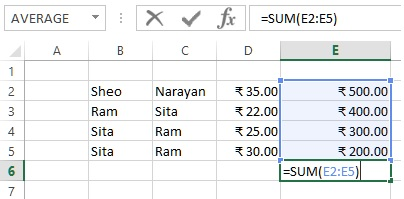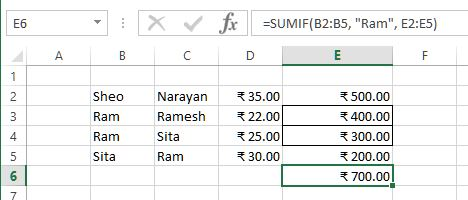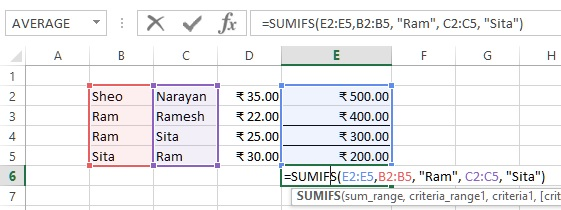# Sum, SumIf, SumIfs functions in Excel

##### How to use Sum, SumIf, SumIfs function in Excel?

In the previous post, we learnt about counting logical and bit values in Excel. In this post, we shall learn about sum range of cells value in Excel.

### Sum function

To sum/add a range of cells in MS Excel, we can use SUM function. SUM function can either by used by directly writing in the cell or by clicking on Sum command from the 'Editing' group under 'HOME' tab on the Ribbon.

So the formula to sum E2 to E5 columns values are '=SUM(E2:E5)'.

Sum by clicking on the RibbonSum by directly writing in the cellHere we are adding the values of E2 to E5 cells and the result is shown below.### SumIf function

Like CountIf function, SUMIF function is also used to sum the range of cells based on one criteria. In below example, we are adding value of E column only when the cell value is more than 350.The criteria is written in double quote as 2nd parameter. Instead of passing 2nd parameter as greater than, less than or equal to, we can also put criteria based on other cell range.

For example, we want to add the E column value only when its corresponding B column value is "Ram". To do this, we have written formula as '=SUMIF(B2:B5, "Ram", E2:E5)' ie. Add the value from E2 to E5 only when B2 to B5 value contains "Ram".and the result is below.### SumIfs function

If our requirement is to use mutilple criteria while summing the range of cells, we can use SumIfs function.

So in below example, we want to sum the value of E column only if B column value is "Ram" and C column value is "Sita". So our formula will be '=SUMIFS(E2:E5, B2:B5, "Ram", C2:C5, "Sita")'.

Here

1. E2 to E5 is sum range
2. B2:B5 is criteria 1 range
3. "Ram" is the criteria value of B2:B5
4. C2:C5 is criteria 2 range
5. "Sita" is the critera value of C2:C5As this criteria is met by only 1 record, so the result is below.Similarly, we can specify more than 2 criteria also to sum range of cells.

Thanks for reading, if this helps do share to your friends and colleagues.

Views: 12212 | Post Order: 19